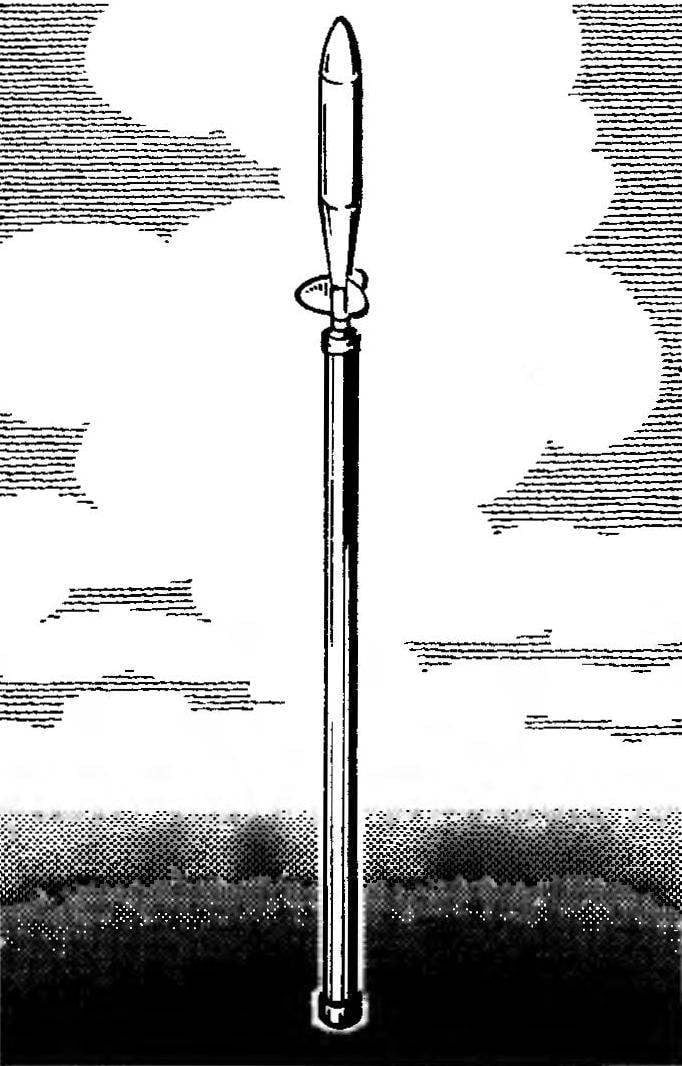# ONCE AGAIN, THE “PISTONE”Article V. Minakova, “Secrets of the new “piston”, published in the “Modeller-designer”, No. 12 1994, aroused great interest among rocketmodeler. It is available for the first time in a popular form expounded basic principles of development and manufacture of gas-dynamic starting unit (GDU).Today we can offer the attention of randomdevice the research summary starts with DGG athletes from the Chelyabinsk region. This report contains calculations of the dynamics of the acceleration of model rockets weighing 230-290 g engines with a total impulse of 20 to 40 N – S. the Authors first examine the effect of parameters where the speed of descent of the model. And the practical value of this material is that the results of the lengthy calculations are given in easy-to-use form.
The main parameters that affect the launch model rockets with gas-dynamic unit (GDU) are the starting mass of the model, engine type and its characteristics, the aerodynamic resistance model and the pipe where the diameter of the piston GDU, elementary zadannyi (recovery) displacement, length acceleration phase, the mass of the pipe where the friction force and the degree of obturation in the system “the piston — pipe” GDU.

Nekotorye of these parameters can be “frozen” within the boundaries of the real nominal values, which can significantly reduce the multiple listing of options for the numerical solution of problems of dynamics mnogoparametricheskikh start. Among the “frozen” parameters, we consider the mass of the movable part of the GDU (50 g), the friction force between the piston and the tube DGG (0.5 N) and the degree of obturation between the piston and the tube GDU (96%).
The calculated structure CDB is shown in figure 1. When you select it was assumed that the friction force in a pair of “motor — hub pipe” is close to zero (loose fit), and the holes in the bottom of the tube provide free drainage of air, eliminating the increase in the pressure in the buffer cavity and the occurrence of the relevant drag forces. The calculation is performed with the following initial data: initial mass of the model 230, 260 and 290 g, aerodynamic resistance model is equivalent to the feathered body of rotation with a length of 800 mm and a diameter of 80 mm, the engine — MRD-or MRD 20-40 at a nominal supply characteristics and a temperature of 20 degrees C, the piston diameter DL = 23.5 mm, the length of the acceleration path Lmax = 700 mm, initial zadannyi (recovery volume) WO = 7.0 noob.cm. On Rianne 2 shows the estimated curve for different values of the starting mass of the model.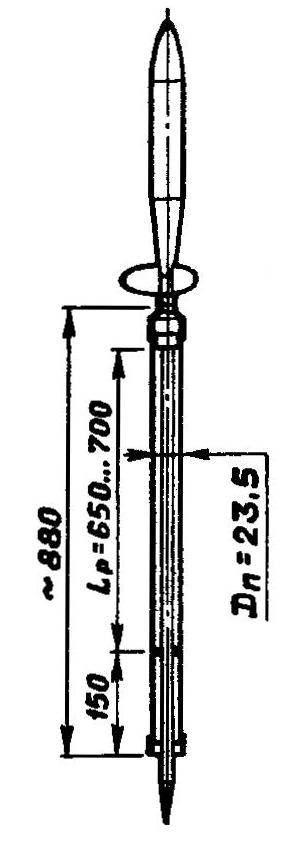Fig. 1. Calculation scheme of the structure of CDB.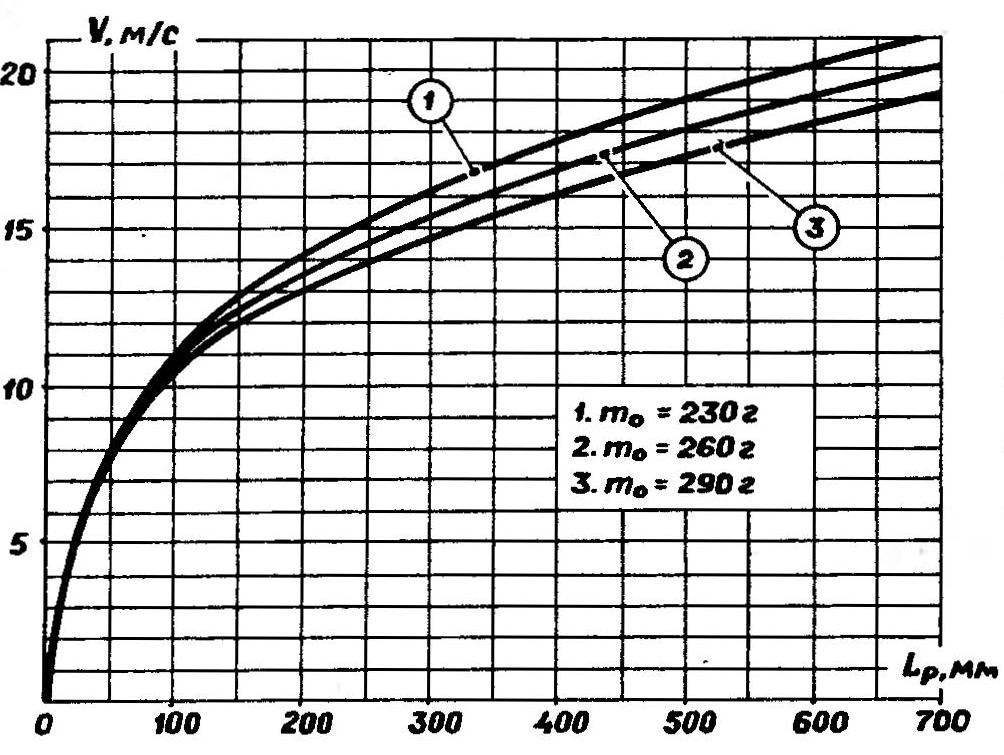Fig. 2. The curve of dependence of speed of descent of the model from the length of the acceleration path.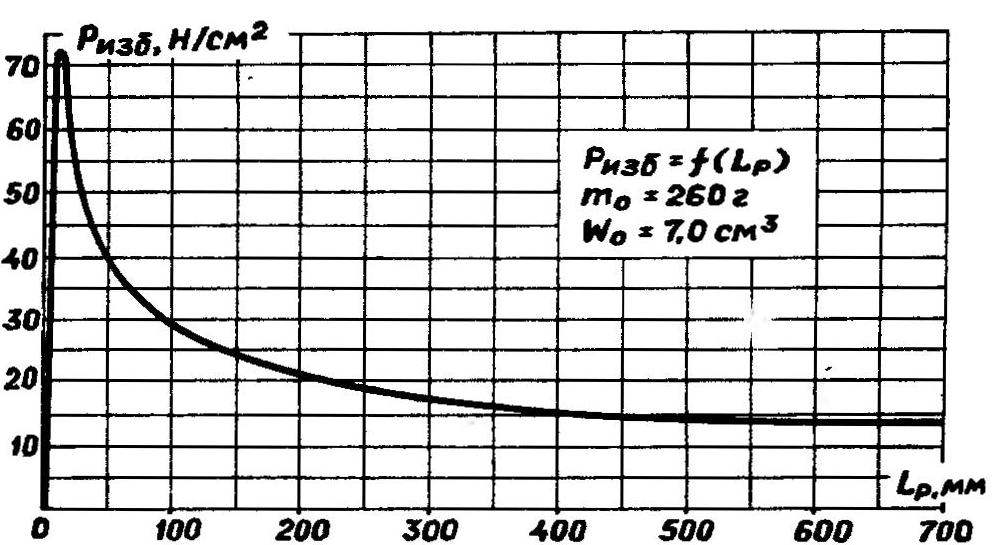Fig. 3. The dependence of the inner excess pressure of the length of the upper area.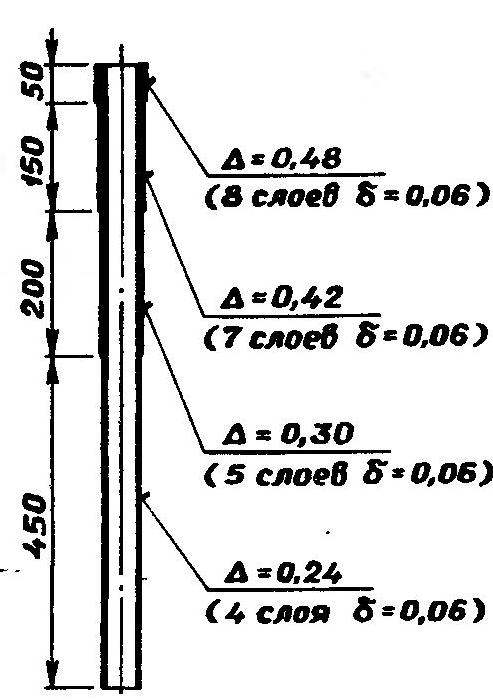Fig. 4. Optimal distribution of wall thickness along the length of the pipe taking into account the actual pressure in the process of acceleration.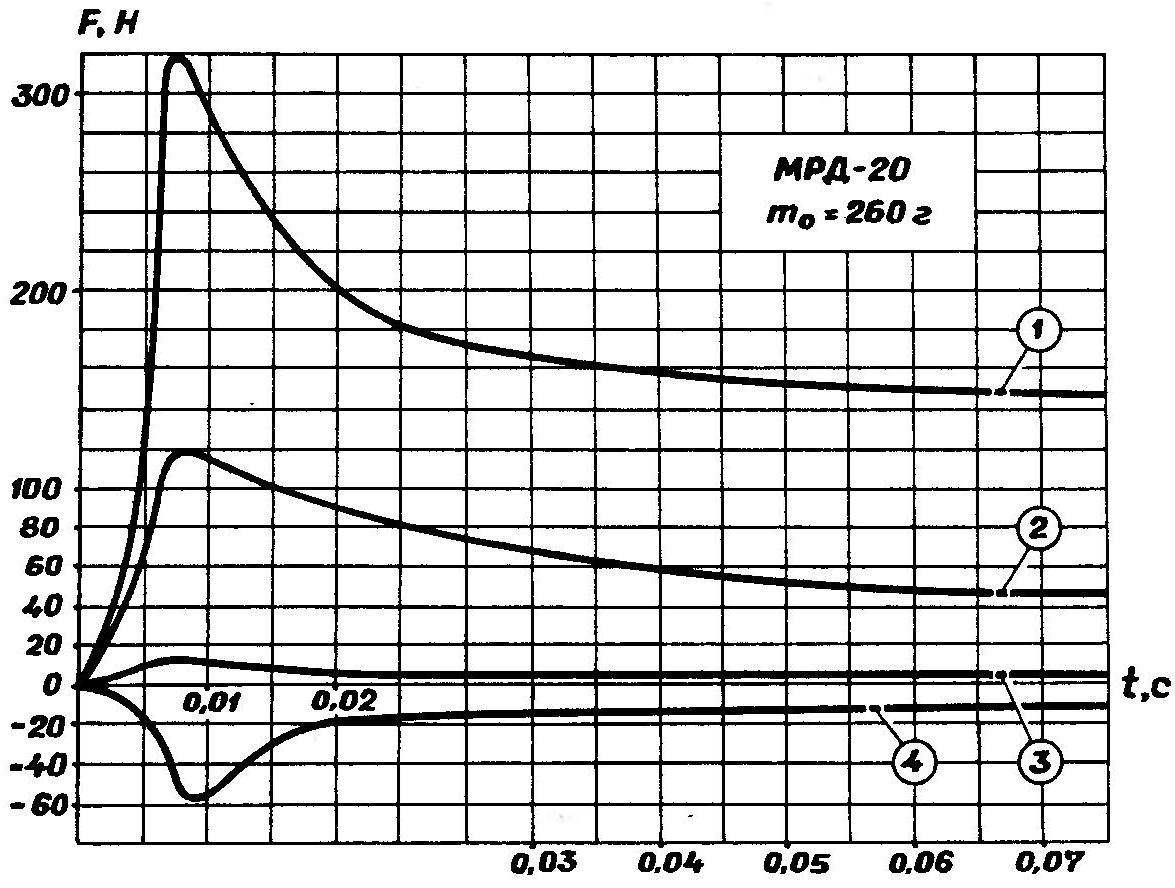Fig. 5.The dependence of total force from the current start time for different values of piston diameter and reviriego volume.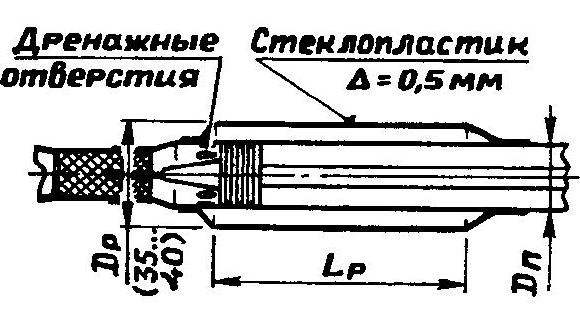Fig. 6. Scheme GDU with pipe reseveral camera.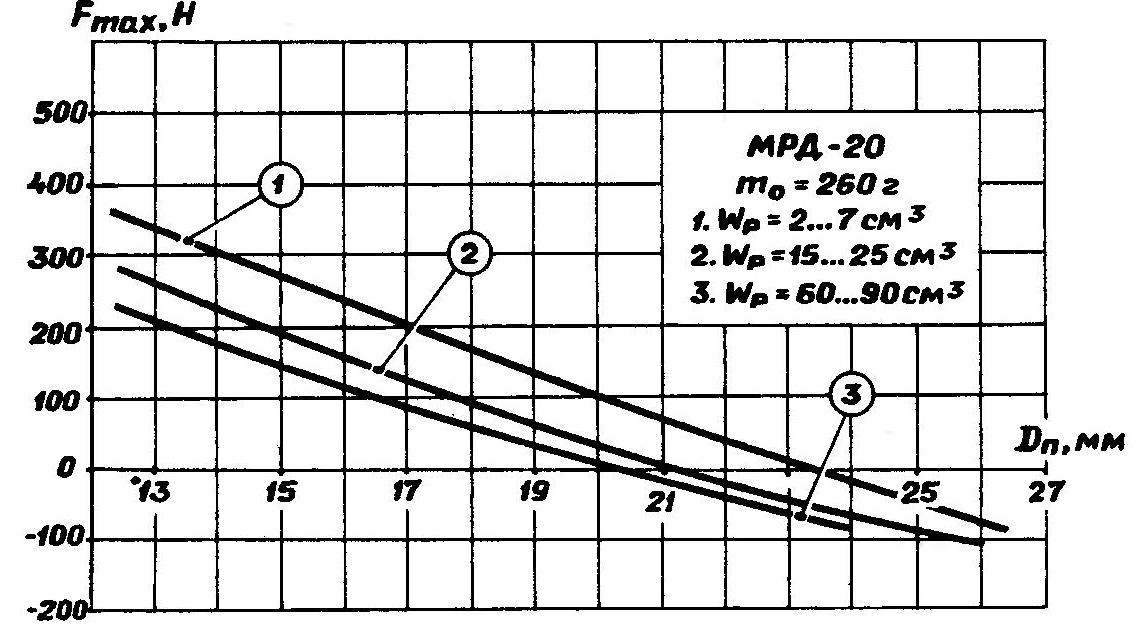Fig. 7. The dependence of the maximum force in planting the “engine hub” of the diameter of the piston and resiverov volume.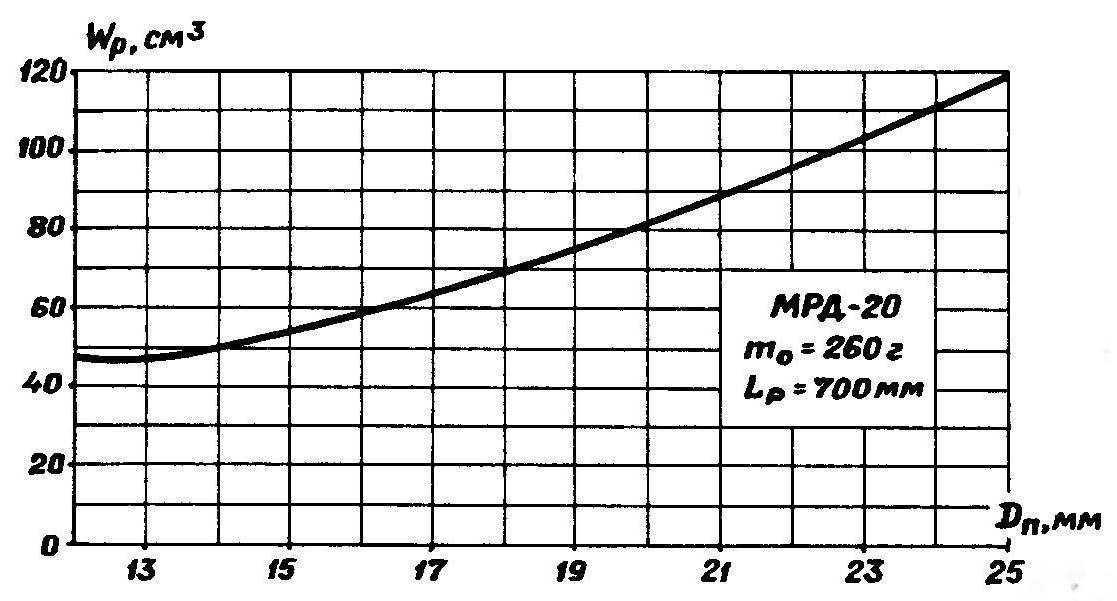Fig. 9. The dependence of the optimal amount recovernow camera WP from the piston diameter for models with a mass of 260 g at the length of the upper area of 700 mm.

The determining parameter for the thickness Stanny pipe is the magnitude of the internal excess pressure of gases flowing from the engine raketnogo in the cavity. The calculated dependence of the inner excess pressure in the tube in the process of acceleration in relation to the model weight of 260 g is shown in figure 3.

With regard to manufacturing technology, to improve the durability of the starting ustanoveni and reduce the number of replacement tubes can be recommended to do strengthening stekloplastikovyh pipe retrieves an additional one or two layers sanatani thickness of 0.06 — 0.1 mm. the Cycle of operation of such products increases in neskolno times with a slight decrease in the rate of descent of the model with GDU. For record-breaking flights and the responsible competition it is better to make the shell of pipe with a variable cross section Stanny in accordance with risunkom 4.
Among the problems determining the operational reliability of the GDU, select the required landing force between the engine and the barrel of the pipe. The solution of this problem allows to exclude premature “jumping” Raney of the sleeve when the engine is started. At the start of the model, this effort should slightly exceed the largest modulus, the maximum force generated in the pair “model — pipe GDU”. The current in the landing zone of the engine and the bushing force can be determined by dependencies: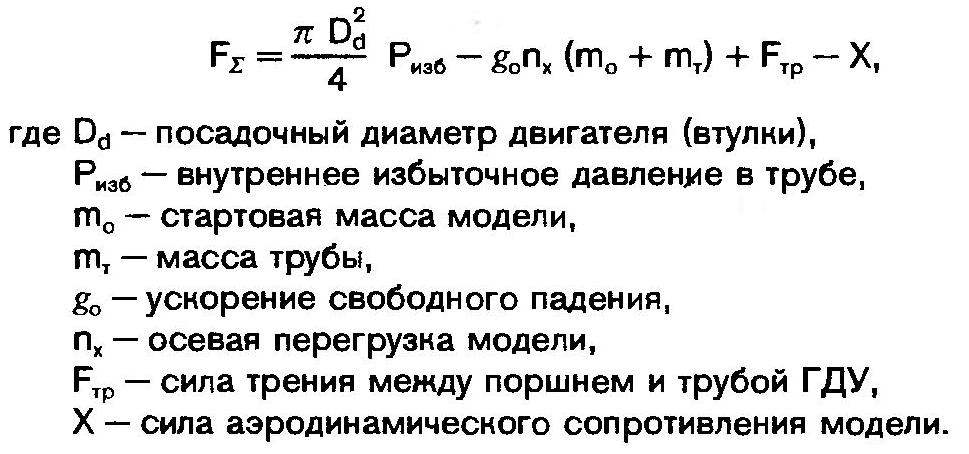Figure 5 graphically shows the results of calculating R for different diameters of piston GDU and sachalinobiini volumes by time of dispersal. Note that when the piston diameter is 23.5 mm and the initial receivername volume Wo = 7 CC maximum force acting on the system “model — pipe GDU” does not exceed 10 N, and so the force of the landing engine in the sleeve pipe may be small, for example 15-20 N.

Figure 6 shows a diagram of the pipe GDU recovernow with the camera. Its volume Wp is determined by the diameters Dp, Dп length Lр. Square drainage holes should be selected with a view to ensuring the free flow of gases from zadannogo volume in the receiver and back. To optimize the proposed scheme, where the calculations of dinamani start for various values of the piston diameter. The results are presented in graphical form in figures 7, 8, 9. From these graphs it follows that in the absence of special recovernow camera the optimal diameter of the piston must be equal to 23.5 mm. While the length of the accelerating portion of the path Lр = 700 mm is achieved the model’s speed of 19.5 m/s. Introduction special recovernow a chamber volume of 80 CC and 90 cm and the diameter reduction of the piston to the optimum value Dn = 20.5 mm will increase the speed of gathering models to 23.5 m/s.

In the development of the CDB and the choice of engine type must be considered that a significant effect on the dynamics of the start model has a flow characteristic of the engine. Responsible for competition and record flights you want to develop a special engine with high flow characteristic.

V. ISAEV, Mikhail POTUPCHIK, A. SEMENOV, G. M a s s Chelyabinsk region.